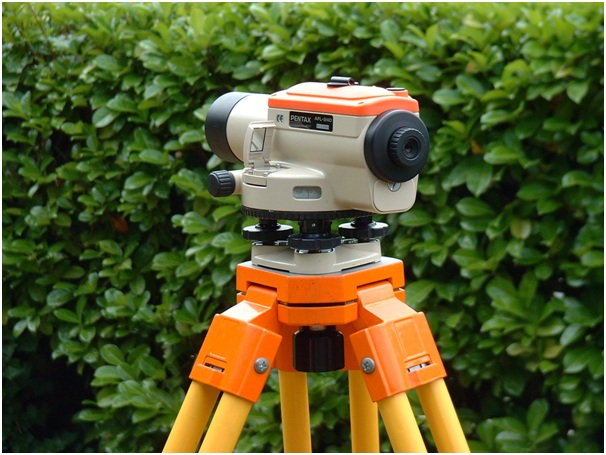Search

# Levelling | Purpose | Terminologies

Posted in Surveying |Email This Post |

Keywords: Levelling, Surveying, Dumpy level, benchmark, Mean Sea Level, M.S.L, Elevation, G.T.S benchmarks, arbitrary benchmarks, datum, Bombay port, Reduced level, Survey of India

What is Levelling?
Levelling is defined as the process of determining the relative heights or elevations of points or objects on the earth’s surface. The elevation of a point is defined as its vertical distance measured above or below a reference level which is called datum. The most widely used datum surface is the Mean Sea Level, M.S.LLevelling instrument: Dumpy level (Source: Wikimedia Commons)

Purpose of leveling
Leveling is done for all important engineering works and construction projects. Some of the purposes for which leveling is done are listed below:

• To find the elevations of points on the earth’s surface for topographic maps.
• For design of highways,railways,canals,sewers etc.
• For laying out of construction projects
• For locating excavating levels
• To determine the drainage characteristics of an area
• Determination of volumes of earthwork for roads,railways etc.

Terminologies in Levelling

• A vertical line is the line indicated by a freely suspended plumb bob or is the line along the direction of gravity.
• Level surface is a surface normal to the plumb line at all points(or perpendicular to the direction of gravity at every point). Simply, we can define it as, any surface that is parallel to the mean spheroidal surface of the earth.
• Mean Sea Level, M.S.L at a location is obtained by averaging the height of the surface of the sea for all stages of the tides for a long period (usually 19 years).
• A level line is defined as any line that is lying on a level surface.
• A datum surface or datum is any arbitrarily assumed level surface from which vertical distances are measured. In India, the datum is the mean sea level at Bombay Port (previously it was the M.S.L at Karachi)
• Elevation of a point(also called the reduce level) is its vertical distance above or below the datum. The Reduced Level (RL) of a point is plus or minus according as the point is above or below the datum.
• The difference in elevation (H)between two points is the vertical distance between level surfaces passing through the two points.
• A horizontal plane through a point is aplane tangential to the level surface at that point. The horizontal planes at different points of level surface are different.
• Horizontal line is any line lying in the horizontal plane
• A vertical plane is a plane containing the vertical line.
• Vertical angle is the angle between two intersecting lines in a vertical plane. The vertical angle of a line is usually measured with respects to a horizontal line intersecting it.The vertical angle is an angle of elevation (if measured above the horizontal line), and an angle of depression (if measured below the horizontal line).
• A benchmark (B.M) is a fixed reference point of known elevation above the datum. A point whose elevation is definitely known can be used as a benchmark. Normally a permanent object is chosen.

Different types of BMs are established and used depending on the permanency and the precision required. They are as follows:

• G.T.S Benchmark or the Great Trigonometric Survey benchmarks are established by the Survey of India throughout the country. The elevation of the benchmarks is correct to two decimal placesof a meter. The level of G.T.S benchmarks is determined very accurately with respect to the M.S.L at Bombay Port.
• Permanent benchmarks are established at closer intervals between widely spread GTS benchmarks. The survey of India or some other government agency such as the PWD established these benchmarks. These are less accurate that G.T.S benchmarks. The permanent benchmarks are marked on aplane surface in the shape of a rectangle. The letter BM and RL are cut and filled in Japan black paint.
• Temporary benchmarks are established temporarily whenever required. These are generally points at which a day’s work is closed and from which the next day’s work is started. They should be carefully established (must be a permanent and stable point) and described in the field book.
• Arbitrary benchmarks are benchmarks whose elevations are arbitrarily assumed for leveling of a small area. The elevations assumed do not refer to any fixed datum such as the M.S.L.

Most of the leveling work can be done assuming an arbitrary benchmark. This is because for most of the applications only the difference of elevations is needed. The point chosen for an arbitrary benchmark should be chosen such that none of the RLs become negative, which leads to confusion in the calculation.

More Entries :